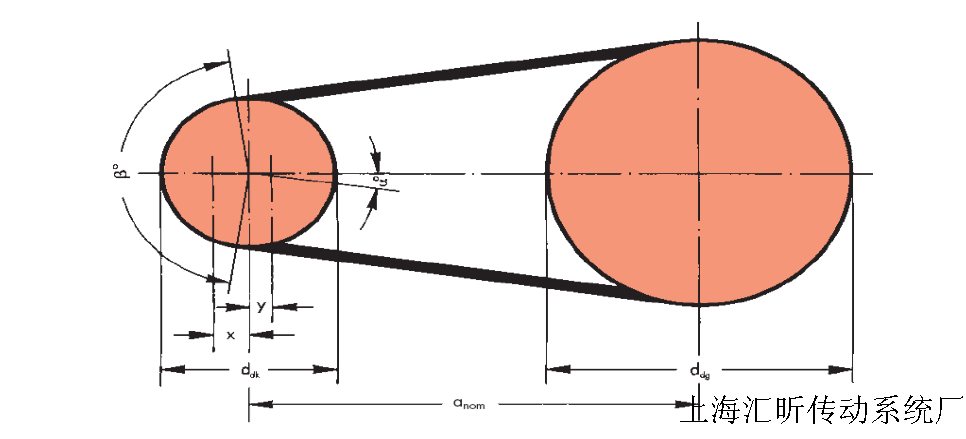•• 1/1
•# 盖茨​​​​三角带 同步带设计计算公式中使用的缩写词

a = 传动中心距（临时） (mm)

anom = 使用标准皮带长度计算的中心距 (mm)

bd = 基准宽度 (mm)

b1 = 顶宽 (mm)

c1 = 包角弧修正系数

c2 = 服务系数

c3 = 带长系数

c4 = 惰轮数量

ddg = 大带轮的基准直径（依据 BS 3790/DIN 2211 选择） (mm)

ddk = 小带轮的基准直径（依据 BS 3790/DIN 2211 选择） (mm)

dd1 = 主动带轮的基准直径 (mm)

dd2 = 从动带轮的基准直径 (mm)

E = 100 mm 跨度上的带偏差 (mm)

Ea = 给定跨度长度的带偏差 (mm)

f = 用于设置皮带张力的负载 (N)

fB = 挠曲比 (s-1)i = 传动比

k = 用于计算带组中离心力的常数

L = 跨度长度 (mm)

List = 标准的内侧带长 (mm)

Lith = 计算所得的内侧带长 (mm)

Ldst = 标准带基准长度 (mm)

Ldth = 计算出的带基准长度 (mm)

ng = 大带轮的转速 (min-1)

nk = 小带轮的转速 (min-1)

n1 = 主动带轮的转速 (min-1)

n2 = 从动带轮的转速 (min-1)

P = 电机功率或额定运转功率 (kW*)

PB = 设计功率 (kW*)

PN = 每条带的额定功率 (kW*)

Sa = 最小静态轴负载 (N)

T = 每条带的最小静态张力 (N)

v = 带速 (m/s)

Ud = 基准周长

x = 带拉伸长和磨损超过中心距 anom的最小公差(mm)

y = 带安装低于中心距 anom的最小公差 (mm)

z = 带的数量

α = 皮带传动装置的角度=900 -2β(°)

β = 小带轮上的包角弧 (°) *1 Kw=1 kNm/s